xGroup velocityEncyclopedia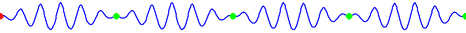The group velocity of a wave
Wave
In physics, a wave is a disturbance that travels through space and time, accompanied by the transfer of energy.Waves travel and the wave motion transfers energy from one point to another, often with no permanent displacement of the particles of the medium—that is, with little or no associated mass...

is the velocity
Velocity
In physics, velocity is speed in a given direction. Speed describes only how fast an object is moving, whereas velocity gives both the speed and direction of the object's motion. To have a constant velocity, an object must have a constant speed and motion in a constant direction. Constant ...

with which the overall shape of the wave's amplitudes — known as the modulation or envelope of the wave — propagates through space.

For example, imagine what happens if a stone is thrown into the middle of a very still pond. When the stone hits the surface of the water, a circular pattern of waves appears. It soon turns into a circular ring of waves with a quiescent center. The ever expanding ring of waves is the wave group, within which one can discern individual wavelets of differing wavelengths traveling at different speeds. The longer waves travel faster than the group as a whole, but they die out as they approach the leading edge.
The shorter waves travel slower and they die out as they emerge from the trailing boundary of the group.

### Definition

The group velocity vg is defined by the equation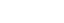where:
ω is the wave's angular frequency
Angular frequency
In physics, angular frequency ω is a scalar measure of rotation rate. Angular frequency is the magnitude of the vector quantity angular velocity...

(usually expressed in radians per second);
k is the angular wavenumber (usually expressed in radians per meter).

The function
Function (mathematics)
In mathematics, a function associates one quantity, the argument of the function, also known as the input, with another quantity, the value of the function, also known as the output. A function assigns exactly one output to each input. The argument and the value may be real numbers, but they can...

ω(k), which gives ω as a function of k, is known as the dispersion relation
Dispersion relation
In physics and electrical engineering, dispersion most often refers to frequency-dependent effects in wave propagation. Note, however, that there are several other uses of the word "dispersion" in the physical sciences....

. If ω is directly proportional
Proportionality (mathematics)
In mathematics, two variable quantities are proportional if one of them is always the product of the other and a constant quantity, called the coefficient of proportionality or proportionality constant. In other words, are proportional if the ratio \tfrac yx is constant. We also say that one...

to k, then the group velocity is exactly equal to the phase velocity
Phase velocity
The phase velocity of a wave is the rate at which the phase of the wave propagates in space. This is the speed at which the phase of any one frequency component of the wave travels. For such a component, any given phase of the wave will appear to travel at the phase velocity...

. Otherwise, the envelope of the wave will become distorted as it propagates. This "group velocity dispersion" is an important effect in the propagation of signals through optical fiber
Optical fiber
An optical fiber is a flexible, transparent fiber made of a pure glass not much wider than a human hair. It functions as a waveguide, or "light pipe", to transmit light between the two ends of the fiber. The field of applied science and engineering concerned with the design and application of...

s and in the design of high-power, short-pulse lasers.

Note: The above definition of group velocity is only useful for wavepackets, which is a pulse that is localized in both real space and frequency space. Because waves at different frequencies propagate at differing phase velocities in dispersive media, for a large frequency range (a narrow envelope in space) the observed pulse would change shape while traveling, making group velocity an unclear or useless quantity.

### Physical interpretation

The group velocity is often thought of as the velocity at which energy
Energy
In physics, energy is an indirectly observed quantity. It is often understood as the ability a physical system has to do work on other physical systems...

or information
Physical information
In physics, physical information refers generally to the information that is contained in a physical system. Its usage in quantum mechanics In physics, physical information refers generally to the information that is contained in a physical system. Its usage in quantum mechanics In physics,...

is conveyed along a wave. In most cases this is accurate, and the group velocity can be thought of as the signal velocity
Signal velocity
The signal velocity is the speed at which a wave carries information. It describes how quickly a message can be communicated between two separated parties...

of the wave
Wave
In physics, a wave is a disturbance that travels through space and time, accompanied by the transfer of energy.Waves travel and the wave motion transfers energy from one point to another, often with no permanent displacement of the particles of the medium—that is, with little or no associated mass...

form. However, if the wave is travelling through an absorptive medium, this does not always hold. Since the 1980s, various experiments have verified that it is possible for the group velocity of laser
Laser
A laser is a device that emits light through a process of optical amplification based on the stimulated emission of photons. The term "laser" originated as an acronym for Light Amplification by Stimulated Emission of Radiation...

light pulses sent through specially prepared materials to significantly exceed the speed of light
Speed of light
The speed of light in vacuum, usually denoted by c, is a physical constant important in many areas of physics. Its value is 299,792,458 metres per second, a figure that is exact since the length of the metre is defined from this constant and the international standard for time...

in vacuum. However, superluminal communication is not possible in this case, since the signal velocity remains less than the speed of light. It is also possible to reduce the group velocity to zero, stopping the pulse, or have negative group velocity, making the pulse appear to propagate backwards. However, in all these cases, photons continue to propagate at the expected speed of light in the medium.

Anomalous dispersion happens in areas of rapid spectral variation with respect to the refractive index. Therefore, negative values of the group velocity will occur in these areas. Anomalous dispersion plays a fundamental role in achieving backward propagating and superluminal light. Anomalous dispersion can also be used to produce group and phase velocities that are in different directions. Materials that exhibit large anomalous dispersion allow the group velocity of the light to exceed c and/or become negative.

### History

The idea of a group velocity distinct from a wave's phase velocity
Phase velocity
The phase velocity of a wave is the rate at which the phase of the wave propagates in space. This is the speed at which the phase of any one frequency component of the wave travels. For such a component, any given phase of the wave will appear to travel at the phase velocity...

was first proposed by W.R. Hamilton
William Rowan Hamilton
Sir William Rowan Hamilton was an Irish physicist, astronomer, and mathematician, who made important contributions to classical mechanics, optics, and algebra. His studies of mechanical and optical systems led him to discover new mathematical concepts and techniques...

in 1839, and the first full treatment was by Rayleigh
John Strutt, 3rd Baron Rayleigh
John William Strutt, 3rd Baron Rayleigh, OM was an English physicist who, with William Ramsay, discovered the element argon, an achievement for which he earned the Nobel Prize for Physics in 1904...

in his "Theory of Sound" in 1877.

### Other expressions

For light, the refractive index n, vacuum wavelength λ0, and wavelength in the medium λ, are related by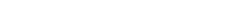The group velocity, therefore, satisfies: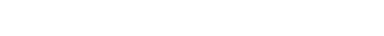## Matter-wave group velocity

Albert Einstein
Albert Einstein
Albert Einstein was a German-born theoretical physicist who developed the theory of general relativity, effecting a revolution in physics. For this achievement, Einstein is often regarded as the father of modern physics and one of the most prolific intellects in human history...

first explained the wave–particle duality
Wave–particle duality
Wave–particle duality postulates that all particles exhibit both wave and particle properties. A central concept of quantum mechanics, this duality addresses the inability of classical concepts like "particle" and "wave" to fully describe the behavior of quantum-scale objects...

of light in 1905. Louis de Broglie hypothesized that any particle should also exhibit such a duality. The velocity of a particle, he concluded then (but may be questioned today, see above), should always equal the group velocity of the corresponding wave. De Broglie deduced that if the duality equations already known for light were the same for any particle, then his hypothesis would hold. This means that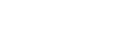where
E is the total energy of the particle,
p is its momentum
Momentum
In classical mechanics, linear momentum or translational momentum is the product of the mass and velocity of an object...

,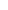is the reduced Planck constant.

For a free non-relativistic particle it follows that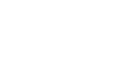whereis the mass
Mass
Mass can be defined as a quantitive measure of the resistance an object has to change in its velocity.In physics, mass commonly refers to any of the following three properties of matter, which have been shown experimentally to be equivalent:...

of the particle andits velocity.

Also in special relativity
Special relativity
Special relativity is the physical theory of measurement in an inertial frame of reference proposed in 1905 by Albert Einstein in the paper "On the Electrodynamics of Moving Bodies".It generalizes Galileo's...

we find thatwhereis the rest mass of the particle,
c is the speed of light
Speed of light
The speed of light in vacuum, usually denoted by c, is a physical constant important in many areas of physics. Its value is 299,792,458 metres per second, a figure that is exact since the length of the metre is defined from this constant and the international standard for time...

in a vacuum,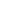is the Lorentz factor
Lorentz factor
The Lorentz factor or Lorentz term appears in several equations in special relativity, including time dilation, length contraction, and the relativistic mass formula. Because of its ubiquity, physicists generally represent it with the shorthand symbol γ . It gets its name from its earlier...

.
and v is the velocity of the particle regardless of wave behavior.

Group velocity (equal to an electron's speed) should not be confused with phase velocity
Phase velocity
The phase velocity of a wave is the rate at which the phase of the wave propagates in space. This is the speed at which the phase of any one frequency component of the wave travels. For such a component, any given phase of the wave will appear to travel at the phase velocity...

(equal to the product of the electron's frequency multiplied by its wavelength).

Both in relativistic and non-relativistic quantum physics, we can identify the group velocity of a particle's wave function with the particle velocity. Quantum mechanics
Quantum mechanics
Quantum mechanics, also known as quantum physics or quantum theory, is a branch of physics providing a mathematical description of much of the dual particle-like and wave-like behavior and interactions of energy and matter. It departs from classical mechanics primarily at the atomic and subatomic...

has very accurately demonstrated this hypothesis, and the relation has been shown explicitly for particles as large as molecules.

• Wave propagation
Wave propagation
Wave propagation is any of the ways in which waves travel.With respect to the direction of the oscillation relative to the propagation direction, we can distinguish between longitudinal wave and transverse waves....

• Dispersion (optics)
Dispersion (optics)
In optics, dispersion is the phenomenon in which the phase velocity of a wave depends on its frequency, or alternatively when the group velocity depends on the frequency.Media having such a property are termed dispersive media...

for a full discussion of wave velocities
• Phase velocity
Phase velocity
The phase velocity of a wave is the rate at which the phase of the wave propagates in space. This is the speed at which the phase of any one frequency component of the wave travels. For such a component, any given phase of the wave will appear to travel at the phase velocity...

• Front velocity
Front velocity
In physics, front velocity is the speed at which the first rise of a pulse above zero moves forward.In mathematics, it is also used to describe the velocity of a possibly propagating front in the solution of hyperbolic partial differential equation....

• Group delay and phase delay
Group delay and phase delay
Group delay is a measure of the time delay of the amplitude envelopes of the various sinusoidal components of a signal through a device under test, and is a function of frequency for each component...

• Signal velocity
Signal velocity
The signal velocity is the speed at which a wave carries information. It describes how quickly a message can be communicated between two separated parties...

• Slow light
Slow light
Slow light is the propagation of an optical pulse or other modulation of an optical carrier at a very low group velocity. Slow light occurs when a propagating pulse is substantially slowed down by the interaction with the medium in which the propagation take place.Researchers at the Rowland...

• Wave propagation speed

• Greg Egan
Greg Egan
Greg Egan is an Australian science fiction author.Egan published his first work in 1983. He specialises in hard science fiction stories with mathematical and quantum ontology themes, including the nature of consciousness...

has an excellent Java applet on his web site that illustrates the apparent difference in group velocity from phase velocity
Phase velocity
The phase velocity of a wave is the rate at which the phase of the wave propagates in space. This is the speed at which the phase of any one frequency component of the wave travels. For such a component, any given phase of the wave will appear to travel at the phase velocity...

.
• Group and Phase Velocity - Java applet with configurable group velocity and frequency.
• Maarten Ambaum has a webpage with movie demonstrating the importance of group velocity to downstream development of weather systems.